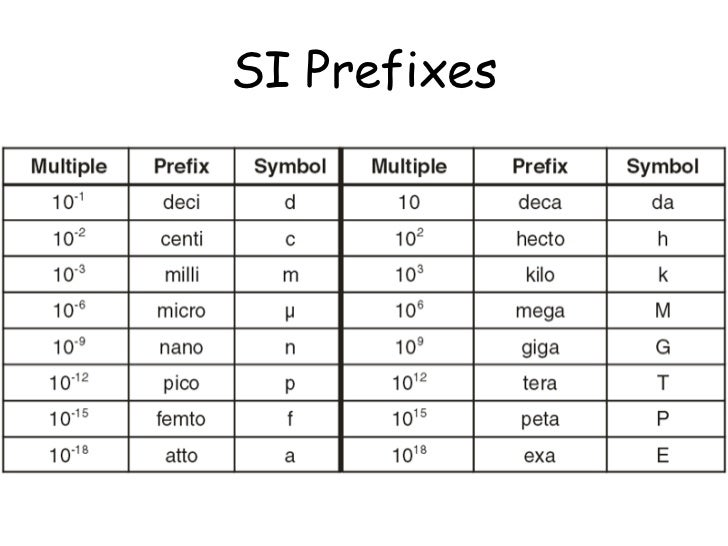# Physics OCR Chapter 2 (Foundations of Physics)

?

## Quantities and Units

Quantity - Is a property of an object or a phenomenon that can be measured

Scientists use a standard system of units abbreviated to SI

SI Base Units

SI is built around seven base units, which are the standard set of units1 of 4

## Prefixes

Prefixes

SI uses prefixes to show multiples of factors of the units.

e.g. km = Kilometer  (The prefix is 'Kilo' the SI base unit is 'meter')2 of 4

## Scalar and Vector Quantities

Scalar Quantities Has a magnitude but no direction

• Scalar quantities can be added and subtracted together in the usual way
• Scalar quantities must have the same units when added or subtracted
• Scalar quantities can also be divided or multiplied together and the units can be the same or different, final units have to be worked out

Vector Quantities  Has magnitude and direction

• Vectors in parallel and antiparallel are added
• Perpendicular Vectors:
• Perpendicular vectors act at right angles to one another
• You can use Pythagoras theorem to find magnitude of the resultant vector
• You can use trigonometry to find the angle
3 of 4

## Resolving Vectors

Resolving Vectors into Two Components

• Can be done using a scale diagram
• Easier to use equation
• A force can be split into two parts the x and y directions
• The equations are:
• F x = F Cos X
• F y = F Cos X
4 of 4